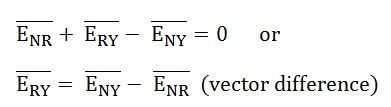# Star Connection in a 3 Phase System

In the Star Connection, the similar ends (either start or finish) of the three windings are connected to a common point called star or neutral point. The three-line conductors run from the remaining three free terminals called line conductors.

The wires are carried to the external circuit, giving three-phase, three-wire star connected systems. However, sometimes a fourth wire is carried from the star point to the external circuit, called neutral wire, forming three-phase, four-wire star connected systems.

Contents:

The star connection is shown in the diagram below:Considering the above figure, the finish terminals a2, b2, and c2 of the three windings are connected to form a star or neutral point. The three conductors named as R, Y and B run from the remaining three free terminals as shown in the above figure.

The current flowing through each phase is called Phase current Iph, and the current flowing through each line conductor is called Line Current IL. Similarly, the voltage across each phase is called Phase Voltage Eph, and the voltage across two line conductors is known as the Line Voltage EL.

## Relation Between Phase Voltage and Line Voltage in Star Connection

The Star connection is shown in the figure below:As the system is balanced, a balanced system means that in all the three phases, i.e., R, Y and B, the equal amount of current flows through them. Therefore, the three voltages ENR, ENY and ENB are equal in magnitude but displaced from one another by 120° electrical.

The Phasor Diagram of Star Connection is shown below:The arrowheads on the EMFs and current indicate direction and not their actual direction at any instant.

Now,There are two-phase voltages between any two lines.

Tracing the loop NRYNTo find the vector sum of ENY and –ENR, we have to reverse the vector ENR and add it with ENY as shown in the phasor diagram above.

Therefore,Similarly,Hence, in star connection line voltage is root 3 times of phase voltage.

## Relation Between Phase Current and Line Current in Star Connection

The same current flows through phase winding as well as in the line conductor as it is connected in series with the phase winding.Where the phase current will be:The line current will be:Hence, in a 3 Phase system of star connections, the line current is equal to phase current.